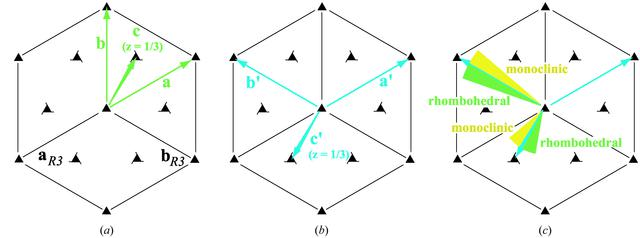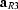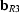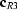disable zoom     view article Figure 6 (a) Symmetry diagram for rhombohedral space group R3, showing the conventionalandunit-cell axes in black. Theaxis is perpendicular to the page. Case (i): axes (a, b, c) of the Niggli (reduced) cell shown in green, with the reduced c axis vector ending at a fractional position z = 1/3 above the plane of the page. (b) Case (ii), an alternate basis set [a′ = a, b′ = b − a, c′ = c – 2(a + b)/3], equally likely to be chosen [equation (6)]. Cell reduction transforms (a′, b′, c′) back into the Niggli cell (a, b, c). (c) Mathematical perturbation or experimental uncertainty in the case (ii) b′ or c′ basis vectors breaks the symmetry, with the (a′, b′, c′) basis no longer reducing to (a, b, c) when either b′ or c′ is perturbed clockwise. If method A (§2.8) is then used to compute the metric symmetry, the result incorrectly depends on the sign of the perturbation.JOURNAL OFAPPLIEDCRYSTALLOGRAPHY
ISSN: 1600-5767
Volume 37| Part 3| May 2004| Pages 399-409